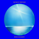7551 lượt xem
Moving Regression is a generalization of moving average and polynomial regression.

The procedure approximates a specified number of prior data points with a polynomial function of a user-defined degree. Then, polynomial interpolation of the last data point is used to construct a Moving Regression time series.

Application:
Moving Regression allows one to smooth noise on the analyzed chart, assess momentum, confirm trends, and establish areas of support and resistance .
In addition, it can be used as a simple stand-alone forecasting method to identify trend direction and trend​ reversal points. When the local polynomial is predicted to move up in the next time step, the color of the Moving Regression curve will be green. Otherwise, the color of the curve is red. This function is (de)activated using the Predict Trend Direction flag.

Selecting the ​model parameters:
The effects of the moving window Length and the Local Polynomial Degree are confounded. This allows for​ finding the optimal trade-off between noise (variance) and lag (bias). Higher Length and lower Polynomial Degree (such as 1, i.e. linear), will result in "smoother" time series but at the cost of greater lag. Increasing the Polynomial​ Degree to, for example, 2 (squared) while maintaining the Length will diminish the lag and thus compromise the noise-lag tradeoff.

Relation to other methods:
When the degree of the local polynomial is set to 0 (i.e., fitting data to a constant level), the Moving Regression time series exactly matches the Simple Moving Average of the same length.
Phát hành các Ghi chú: minor corrections
Mã nguồn mở

Với tinh thần của TradingView, tác giả đã xuất bản tập lệnh theo mã nguồn mở, vì thế trader có thể dễ dàng hiểu và tùy chỉnh được. Bạn có thể sử dụng miễn phí, hoặc tùy chỉnh lại mã đã được cấp phép bởi Quy tắc Chung. Bạn có thể sử dụng nó trên biểu đồ.

Bạn muốn sử dụng tập lệnh này trên biểu đồ?

## Bình luậnThis looks like the Least Squares Moving Average
Phản hồijustinsandell
@justinsandell, In my experience, the name "Least Squares Moving Average" is commonly associated with local linear regression. The current script is built on polynomial regression and therefore can be viewed as a generalization. But of course you can achieve the local linear regression by setting Polynomial Degree to 1.
Phản hồiMMC11
@MMC11, the script produces only one line as output, i.e. the MR curve (plotted in green-red color). The blue line that you see in the preview is just the closing price.
Phản hồi